# Steering Behaviors: Wander 漫游

Wander 行为意图产生一种真实可玩的移动行为，使玩家以为游戏AI是真实的生命体在游戏中巡逻。

```// Calculate the wander force
private function wander() :Vector3D {
var now :Number = (new Date()).getTime();

if (now >= nextDecision) {
// Choose a random position for "target"
}

// return a steering force that pushes the character
// towards the target (the seek behavior)
return seek(target);
}

// In the game loop, handle forces and movement just
// like before:
public function update() :void {
steering = wander()
steering = truncate (steering, max_force)
steering = steering / mass
velocity = truncate (velocity + steering , max_speed)
position = position + velocity
}```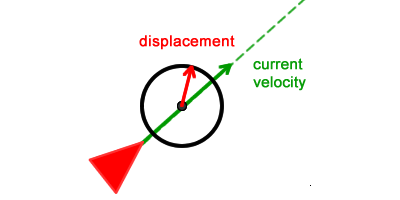```// The CIRCLE_DISTANCE constant below is
// a number defined somewhere else.
// The code to calculate the circle center:
var circleCenter :Vector3D;
circleCenter = velocity.clone();
circleCenter.normalize();
circleCenter.scaleBy(CIRCLE_DISTANCE);```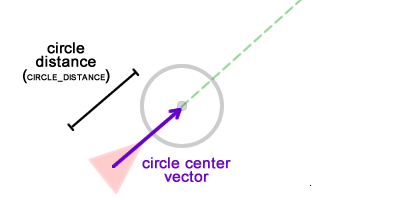```var displacement :Vector3D;
displacement = new Vector3D(0, -1);
//
// Randomly change the vector direction
// by making it change its current angle
setAngle(displacement, wanderAngle);
//
// Change wanderAngle just a bit, so it
// won't have the same value in the
// next game frame.
wanderAngle += (Math.random() * ANGLE_CHANGE) - (ANGLE_CHANGE * .5);```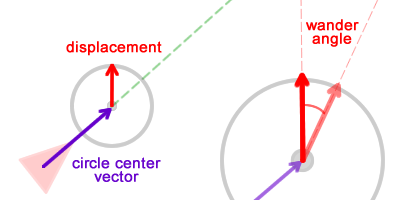```var wanderForce :Vector3D;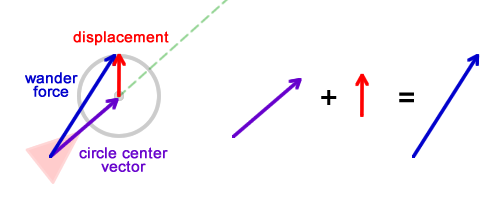wander力可以想象成以游戏AI为起点，指向圈上的某个点向量，具体这个点的位置会决定施加在游戏AI身上的力是朝左还是右，是强还是弱：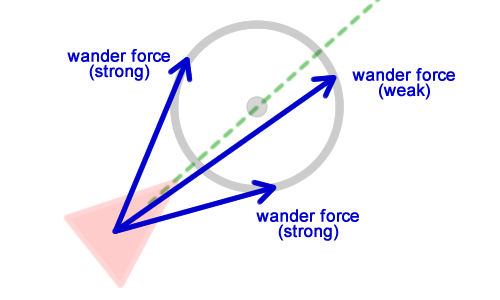wander力越和速度向量平行，游戏AI转变得就越少，wander力和之前介绍的seek和flee力一样，会将游戏AI推向一个方向。但是不同的是前者是根据圈上的一个随机位置来决定推向哪，而后者是根据一个目标位置。

```private function wander() :Vector3D {
var circleCenter :Vector3D;
circleCenter = velocity.clone();
circleCenter.normalize();
circleCenter.scaleBy(CIRCLE_DISTANCE);
var displacement :Vector3D;
displacement = new Vector3D(0, -1);
setAngle(displacement, wanderAngle);
wanderAngle += Math.random() * ANGLE_CHANGE - ANGLE_CHANGE * .5;
var wanderForce :Vector3D;
return wanderForce;
}

public function setAngle(vector :Vector3D, value:Number):void {
var len :Number = vector.length;
vector.x = Math.cos(value) * len;
vector.y = Math.sin(value) * len;
}```

```steering = wander()
steering = truncate (steering, max_force)
steering = steering / mass
velocity = truncate (velocity + steering , max_speed)
position = position + velocity```